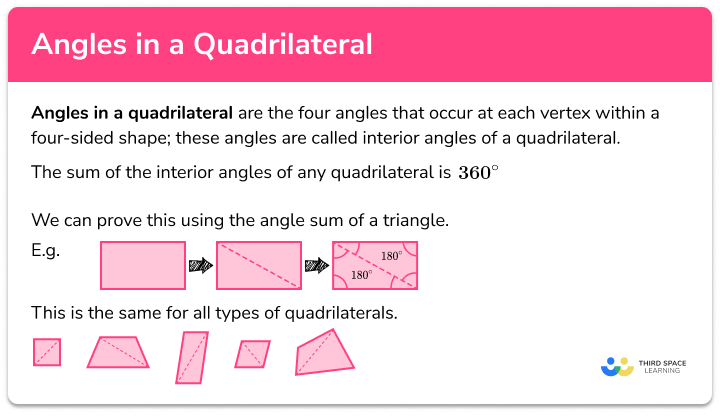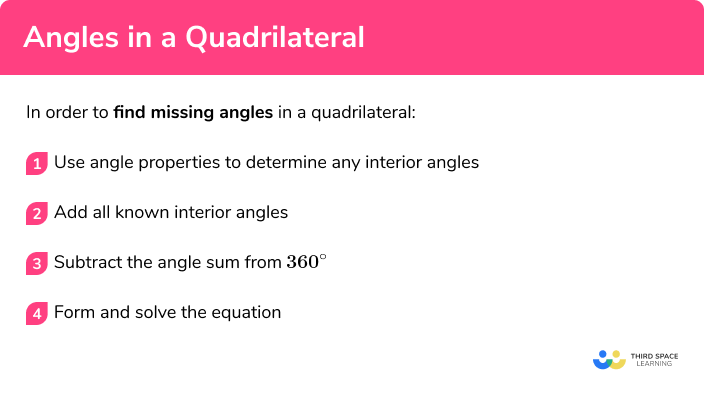Here we will learn about angles in a quadrilateral, including the sum of angles in a quadrilateral, how to find missing angles, and using these angle facts to generate equations and solve problems.

There are also angles in quadrilaterals worksheets based on Edexcel, AQA and OCR exam questions, along with further guidance on where to go next if you’re still stuck.

## What are angles in a quadrilateral?

Angles in a quadrilateral are the four angles that occur at each vertex within a four-sided shape; these angles are called interior angles of a quadrilateral. The sum of the interior angles of any quadrilateral is 360° .

We can prove this using the angle sum of a triangle.

The rectangle above is split into two triangles by joining two vertices together across the diagonal.

As the sum of angles in a triangle is 180° , we can add two lots of 180° together, making the angle sum of a quadrilateral equal to 360° .

This is the same for all types of quadrilaterals.

### What are angles in a quadrilateral?The four angles in any quadrilateral always add to 360° , but there are a few key properties of quadrilaterals that can help us calculate other angles.

Parallelogram:

• Opposite angles are the same (congruent)
• Opposite sides are the same
• Two pairs of supplementary angles (co-interior)
• Vertically opposite angles at the intersection of the diagonals

Rectangle:

• All the properties of a parallelogram and
• All edges meet at right angles

Kite:

• The diagonals are perpendicular lines
• One pair of opposite angles are congruent

Rhombus:

• All properties of a parallelogram and
• All sides are equal in length
• The diagonals 4 congruent triangles

Square:

• All the properties of a rectangle and a rhombus and
• The diagonals form 4 isosceles triangles

Trapezium:

• Angles at the intersection of the diagonals are vertically opposite.
• One pair of parallel sides, therefore two pairs of supplementary angles (co-interior)

Isosceles trapezium:

• All properties of a trapezium and
• Two pairs of congruent angles

• Diagonals bisect at 90 degrees external to the shape (if symmetrical)
• One pair of congruent angles (if symmetrical)
• One reflex interior angle

## How to find missing angles in a quadrilateral

In order to find missing angles in a quadrilateral:

1. Use angle properties to determine any interior angles.
2. Add all known interior angles.
3. Subtract the angle sum from 360° .
4. Form and solve the equation.

### How to find missing angles in a quadrilateral### Related lessons on angles in polygons

Angles in a quadrilateral is part of our series of lessons to support revision on angles in polygons. You may find it helpful to start with the main angles in polygons lesson for a summary of what to expect, or use the step by step guides below for further detail on individual topics. Other lessons in this series include:

## Angles in a quadrilateral examples

### Example 1: trapezium

ABCD is a trapezium. Calculate the size of angle BCD , labelled x :

1. Use angle properties to determine any interior angles.

Angle fact:

The line AD is perpendicular to lines AB and CD so angle BAD = 90° .

90+90+110=290^{\circ}

3Subtract the angle sum from \pmb {360°} .

Here, 360 - 290 = 70°

So, x = 70° .

We could have also found this angle using the fact that angle ABC and angle BCD are co-interior angles and, therefore, must add to 180° .

Find the value of the missing angle x :

Angle fact:

123+77+65=265^{\circ}

Here, 360 - 265 = 95°

So, x = 95° .

### Example 3: parallelogram with one interior angle (form and solve)

Calculate the missing angle for the following parallelogram:

Angle fact:

• Co-interior angles add to equal 180° .

x+32

Here,

### Example 4: parallelogram with one interior angle (form and solve)

Calculate the missing angle for the following parallelogram:

Angle fact:

• Diagonally opposite angles in a parallelogram are equal:

x+32+x+32=2x+64

Here,

### Example 5: exterior angle given

Calculate the missing angle for the following quadrilateral

Angle fact:

• Angles on a straight line add to equal 180° :

99+105+75=279^{\circ}

Here, 360 - 279 = 81°

So, x = 81° .

### Example 6: forming and solving equations

By finding the value for x , calculate the value of each angle in the kite drawn below:

Angle fact:

• One pair of diagonally opposite angles in a kite are the same size:

45+2x+x+2x=5=5x+45

Here,

As x = 63° we can find the value for the remaining angles in the kite by substituting the value onto each angle:

2x = 126°

So we have the four angles: 45°, 126°, 126°, and 63° .

We can check the solution by adding these angles together. They should add to equal 360° .

45 + 126 + 126 + 63 = 360° .

### Example 7: forming and solving equations

Find the value for x , given the values of each angle in the quadrilateral:

For an irregular quadrilateral, there is only one angle property: the sum of the angles is equal to 360° .

100+2x-30+5x-40+3x=10x+110

Here,

### Common misconceptions

• Mistaking the sum of angles in a quadrilateral with the angles in a triangle

The angle sum is remembered incorrectly as 180° , rather than 360° . The sum of angles in a triangle is equal to 180° .

• Join all the diagonals

When recalling the angle sum in a quadrilateral, students join all the diagonals together, creating 4 triangles. This makes their angle sum 720° which is also incorrect.

• Incorrect angle fact

A common mistake is to use the incorrect angle fact or make an incorrect assumption to overcome a problem.

E.g.

Here the trapezium is assumed to be symmetrical (an isosceles trapezium) so the interior angles are easy to deduce. This is not always true and so you should use co-interior angles instead.

Here, the angle x should be equal to 60° and y should be equal to 105° due to co-interior angles in parallel lines.

### Practice angles in a quadrilateral questions

1.  ABCD is a rhombus. Given that CDA = 84^{\circ} calculate the value of a .96^{\circ}84^{\circ}6^{\circ}168^{\circ}Diagonally opposite angles in a rhombus are equal.

Co-interior angles add to equal 180^{\circ} .

180-84=96^{\circ}

2. ABCD is a trapezium. Use the information below to calculate the value of b .89^{\circ}72^{\circ}199^{\circ}91^{\circ}Co-interior angles add to equal 180^{\circ} .
180-89=91^{\circ}

3. ABCD is a parallelogram. Calculate the size of the angle BCD .200^{\circ}40^{\circ}100^{\circ}50^{\circ}5x+4x=180 (co-interior)

9x=180\\ x=20\\ BCD=5x=100^{\circ} .

4. ABCD is a quadrilateral. Given that CE  is a straight line, calculate the interior angle at D marked x .279^{\circ}155^{\circ}223^{\circ}137^{\circ}Angles on a straight line add to equal 180^{\circ} .5. ABCD is an isosceles trapezium. Calculate the value of y .55^{\circ}125^{\circ}155^{\circ}175^{\circ}y=180-(3\times50-25) y=180-125 y=55^{\circ}

6. ABCD is an irregular quadrilateral where BE is a straight line through C . Calculate the exact size of the angle y .84^{\circ}6^{\circ}168^{\circ}100^{\circ}Angles on a straight line add to equal 180^{\circ} .

y=180-(140-2x)=2x+40\\ x+30+x+5x+20+2x+40=9x+90

9x+90=360^{\circ}As x=30^{\circ}, y=2x+40=230+40=100^{\circ} .

### Angles in quadrilaterals GCSE questions

1. Show that the two quadrilaterals below are similar.(5 marks)

Co-interior angles add to equal 180^{\circ}

(1)

ABC=180-116=64180^{\circ} and ABC=EFG

(1)

FGH=180-64=116180^{\circ} and FGH=BCD

(1)

Diagonally opposite angles in a parallelogram are equal

(1)

All angles correspond and the sides are enlarged by a scale factor of 2

(1)

2.

(a) Calculate the size of angle \theta in the trapezium ABCD .(b) What type of trapezium is ABCD ?
(c) State 2 properties about shape ABCD .

(6 marks)

(a)
Angles on a straight line add to equal 180^{\circ} and angle CDA=68^{\circ} .

(1)

Vertically opposite angles are equal and angle BCA=68^{\circ} .

(1)

DAB + CDA = 180^{\circ} because they are co-interior so \theta=112^{\circ}

(1)

(b)
An isosceles trapezium

(1)

(c)
Line AB and CD are parallel

(1)

One line of symmetry

(1)

3. Four matchsticks are dropped on the floor. They make a quadrilateral in the following arrangementUse the information in the diagram to calculate the size of each interior angle of the shaded region.

(5 marks)

Vertically opposite angles are equal

(1)

Angles on a straight line add to equal 180^{\circ}

(1)

(1)

x=17^{\circ}

(1)

Angles: 98^{\circ}, 95^{\circ}, 110^{\circ}, 57^{\circ}

(1)

## Learning checklist

You have now learned how to:

• Find unknown angles in any quadrilateral

## Still stuck?

Prepare your KS4 students for maths GCSEs success with Third Space Learning. Weekly online one to one GCSE maths revision lessons delivered by expert maths tutors.

Find out more about our GCSE maths tuition programme.